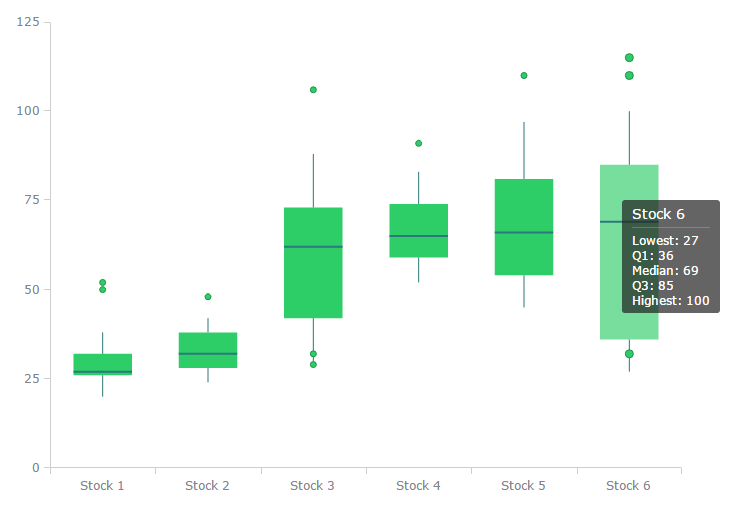# What is a Box Plot and How to Read It

A Box Plot is a convenient way of graphically depicting groups of numerical data through their quartiles. They provide a graphical rendition of statistical data based on the minimum, first quartile, median, third quartile, and maximum, also Outliers can be plotted as individual points.

The term "box plot" comes from the fact that the graph looks like a rectangle with lines extending from the top and bottom. Because of the extending lines, this type of graph is sometimes called a box-and-whisker plot. The distances between the different box parts represent the degree of a data dispersion and a data asymmetry to identify outliers. The points values can be compared between themselves (single-series chart) or values inside the category (multi-series chart). In case of several series points are grouped by categories.### Interpreting a Box Plot

The box plot is interpretted as follows:

• The box itself contains the middle 50% of the data. The upper edge (hinge) of the box indicates the 75th percentile of the data set, and the lower hinge indicates the 25th percentile. The range of the middle two quartiles is known as the inter-quartile range.
• The line in the box indicates the median value of the data.
• If the median line within the box is not equidistant from the hinges, then the data is skewed.
• The ends of the vertical lines or "whiskers" indicate the minimum and maximum data values, unless outliers are present in which case the whiskers extend to a maximum of 1.5 times the inter-quartile range.
• The points outside the ends of the whiskers are outliers or suspected outliers.

Get our Data Science for Finance Bundle for just $29$51.